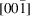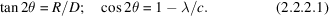International
Tables for
Crystallography
Volume C
Mathematical, physical and chemical tables
Edited by E. Prince

International Tables for Crystallography (2006). Vol. C, ch. 2.2, p. 30

## Section 2.2.2.2. Crystal setting

J. R. Helliwella

aDepartment of Chemistry, University of Manchester, Manchester M13 9PL, England

#### 2.2.2.2. Crystal setting

| top | pdf |

Crystal setting follows the procedure given in Subsection 2.2.1.2whereby angular mis-setting angles are given by equation (2.2.1.3). When viewed down a zone axis, the pattern on a flat film or electronic area detector has the appearance of a series of concentric circles. For example, with the beam parallel to, the first circle corresponds to l = 1, the second to l = 2, etc. The radius of the first circle R is related to the interplanar spacing between the (hk0) and (hk1) planes, i.e. λ/c (in this example), through, by the formulae